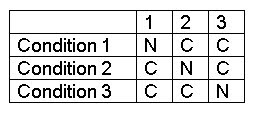How Many Ways to Analyze the Word ‘God’? – Part 3

II. Only Three Attributes are Relevant

In this second case there will be far fewer possible definitions of ‘divine person’ because the assumption that one of the four attributes is irrelevant means that we only have three attributes to use in constructing sets of conditions that will in turn be used to generate definitions. Fewer elements means fewer combinations of elements can be formed.

The following table illustrates the various possibilities in which only three of the four attributes are relevant (R = relevant; I = irrelevant):Since there are only three conditions in each set of conditions, each column in the above table represents 10 x 10 x 10 = 100 x 10 = 1,000 different sets of three conditions. Thus, the four columns of this table represent a total of 4,000 different sets of three conditions.
A. All Three Conditions are Criterial and None are Necessary Conditions
If all three conditions are taken as criterial, then each of the 4,000 sets of conditions is ambiguous. This is because two different strengths of requirement can be imposed on a set of three criterial conditions:
At least two conditions must be satisfied
At least one condition must be satisfied
Therefore, each of the 4,000 sets of three conditions can be used to make two criterial definitions. This means that there are 4,000 sets of conditions x 2 strengths of requirement = 8,000 definitions consisting of three criterial conditions.
B. All Three Conditions are Necessary Conditions and None are Criterial
Since there is just one strength of requirement for definitions that are composed of only necessary conditions (i.e. every condition must be satsified, without exception), the 4,000 sets of three conditions can be used to generate 4,000 definitions that are composed of three necessary conditions.
C. Two Conditions are Criterial and One is a Necessary Condition
When you mix criterial conditions and necessary conditions, they can occur in different orders. With sets of three conditions, there are three different possible permutations consisting of two criteria and one necessary condition (C = criterial condition; N = necessary condition):This means that we can take the 4,000 different sets of three conditions, and generate a total of 12,000 sets of conditions that consist of two criteria and one necessary condition (4,000 sets of conditions x 3 different permutations = 12,000 sets consisting of two criteria and one necessary condition).
There is only one strength requirement that can be used for definitions involving just two criteria:
At least one of the (criterial) conditions must be satisfied

So, we can generate only one defintion from each set of conditions, and thus this subset generates 12,000 definitions that consist of two criteria and one necessary condition.

D. One Condition is Criterial and Two are Necessary Conditions

This subset of definitions is easy to enumerate. There are none, because any correct definition that includes a criterial condition must include at least two criteria.

E. Adding Up the Definitions from each Subset (A-D)

8,000 definitions (consisting of three criterial conditions)
4,000 definitions (consisting of three necessary conditions)
12,000 definitions (consisting of two criteria and one necessary condition)
0 definitions (consisting of one criterial condition and two necessary conditions)

The total number of different definitions of ‘divine person’ that can be generated in the case that only three of the four attributes are relevant is:

24,000 definitions